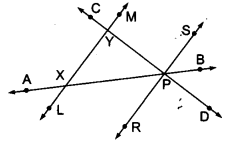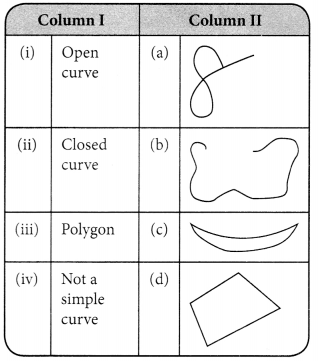# MCQ Questions for Class 6 Maths Chapter 4 Basic Geometrical Ideas with Answers

We have compiled the NCERT MCQ Questions for Class 6 Maths Chapter 4 Basic Geometrical Ideas with Answers Pdf free download covering the entire syllabus. Practice MCQ Questions for Class 6 Maths with Answers on a daily basis and score well in exams. Refer to the Basic Geometrical Ideas Class 6 MCQs Questions with Answers here along with a detailed explanation.

## Basic Geometrical Ideas Class 6 MCQs Questions with Answers

Choose the correct option.

Question 1.
Least number of line segments required to make a polygon is
(a) 1
(b) 2
(c) 3
(d) 4

Question 2.
How many lines can be drawn through given two points?
(a) Only one
(b) 2
(c) 4
(d) Countless

Question 3.
How many vertices are there in a triangle?
(a) 1
(b) 2
(c) 3
(c) 4

Question 4.
Find ‘False’ statement.
(a) Two diameters of a circle will necessarily intersect.
(b) The centre of a circle always lies in the interior.
(c) The diameter is half of the radius of a circle.
(d) Longer chord is nearer to the centre of the circle.

Answer: (c) The diameter is half of the radius of a circle.

Question 5.
Which of the following has two end points?
(a) Line
(b) Ray
(c) Line-segment
(d) None of these

Question 6.
Number of lines which can be drawn passing through two given points:
(a) 1
(b) 2
(c) 3
(d) An infinite number

Fill in the blanksQuestion 1.

Question 2.
……………….. is a diameter of the circle.

Question 3.
……………….. is a chord of the circle.

Question 4.
……………….. is a radius of the circle.

Question 5.
Line segment PO = line segment ……………….. (in length)

Question 6.
PR is dividing the circle into two equal halves, and each half is called ………………..

Q. 7 – 8 Look the picture and answer them.Question 7.
The lines intersecting at P are: ……………., ……………. and …………….

Answer: $$\bar{RS}$$, $$\bar{AB}$$, $$\bar{CD}$$

Question 8.
The concurrent lines are: ……………., ……………. and …………….

Answer: $$\bar{AB}$$, $$\bar{CD}$$, $$\bar{RS}$$

Question 9.
A line segment has ……………. end points(s).

Question 10.
A ray has ……………. endpoint(s).

Question 11.
Two lines intersect at the most at ……………. points

Question 12.
The maximum number of points of intersection of three lines is …………….

State True or False

Question 1.
Sector of a circle is the region in the interior of a circle enclosed by an arc on one side and a pair of radii on the other two sides.

Question 2.
Circle is a polygon.

Question 3.
A line is also a curve.

Question 4.
Interior of an angle is a restricted area.

Question 5.
Line is a part of a ray.

Question 6.
Two lines in a plane can intersect each other at two points.

Question 7.
Line segment is a definite part of a line.

Question 8.
Through a given point, only one line can be drawn.

Match the Following(i) → b
(ii) → c
(iii) → d
(iv) → a

 Column I Column II (a) A line (i) measure (b) A line segment can be (ii) extends infinitely in one direction. (c) A ray (iii) measured (d) In an angle, the lengths of the arms do not affect its (iv) extends infinitely in both directions.